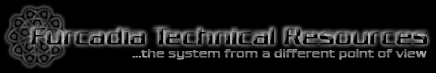Documentations base95 Numbers  Commands  Server Instructions  Colormap  Movement/Coordination  Palette  Entities  Badge Hash-signs  Portrait File Map  Avatar Frames  Static Dreams

 File Formats FSH  FS2  FBJ  MAP  DSB  RCH

 Protocol Specifications Main Server  File Server

### base95 Numeric System

You might have already noticed that Furcadia doesn't use a decimal numeric system to specify coordinates and just about anything else in the protocol. In order to reduce bandwidth usage in favor of those who don't own a fast connection to the server (as well as allowing more users to use the server without causing lag), the system uses base95 numbers instead of base10.

Why? The decimal numeric system only allows 10 digits in total (0 - 9), while in base95 system you can get up as high as 95 digits (0 - 94 [sp - ~]). In other words, in case of decimal numeric system you can fit only 10 numbers in a byte, while base95 allows you to have 95 numbers in one.

Now think what ranges you can get to when you're using two digits. In this case we are talking about 100 numbers (00 - 99) versus 9025 numbers (0 - 9024 [spsp - ~~]) that can go in two bytes. base95 seems more efficient, no? ;)

To explain a bit what are base95 numbers - they are numbers that have 95 digits and each digit is represented as a character from the second "block" of the ASCII table (the characters we can freely type with a keyboard) - starting from the space character (32) and ending with the ~ character (126). If you look carefully at one digit of the base95 number and an ASCII table, you will notice each number is actually a character in the table +32.

### Conversion Between Bases

Obviously, base95 is not the kind of system we're used to count in (along with our computers), so to perform mathematical calculations or just about anything else, we'd need to convert them weird digits into the decimal system. Here is a simple way to convert a two-digit base95 number into a decimal one:

 n = ((byte - 32) * 95) + (byte - 32)

Note that we're normally dealing with unsigned numbers - there should be no negative values in Furcadia's data! Here is a simple equasion to convert a number between 0 and 9024 into a base95 string:

 byte = (n / 95) + 32 byte = (n % 95) + 32

Basicly, that's all to it when it comes to converting between base95 and decimal numbers and since you will most likely not deal with numbers higher than two digits in Furcadia, you wouldn't need fancier conversion algoritm. If you wish to be able to convert at a higher range, have a look below for a pair of functions that do just that.

### Simple Conversion Functions

The functions below are C/C++ compatible and allow base95 conversion between 2-byte strings and numbers from 0 to 9024 - it's pretty much their limit:

 ```/*** String -> Integer ***/ short StoI (const char * byte) { if (((unsigned char) byte < 32) || (unsigned char) byte > 126)) return -1; if (((unsigned char) byte < 32) || (unsigned char) byte > 126)) return -1; return (((unsigned char) byte - 32) * 95) + ((unsigned char) byte - 32)); } /*** Integer -> String ***/ void ItoS (char * byte, short n) { if (n < 0) n = 0; if (n > 9024) n = 9024; byte = (unsigned char)((n / 95) + 32); byte = (unsigned char)((n % 95) + 32); byte = '\0'; }```

The functions here perform the same conversion routines, but their limits are a lot more flexible: they are not limited to 9025 numbers, but can go as far as a long (32bit) number can! They take more resources, so think carefully if you really need to use these...

 ```/*** String -> Integer ***/ long StoI (char * str, unsigned char len) { long n = 0; // Output number int m = 1; while (len > 0) { n += (str[--len] - 32) * m; m *= 95; } return n; } /*** Integer -> String ***/ char * ItoS (char * str, long n) { int len = 0; ItoS_ (str, n, &len); str[len] = '\0'; return str; } /*** ItoS Helper Function ***/ void ItoS_ (char * str, long n, int * len) { if (n > 0) ItoS_ (str, n / 95, len); else return; str[*len] = (n % 95) + 32; // Reverse order *len += 1; }```

### base95 Digit Table

If you can't be bothered doing it on your own, here is a table of digits for the base95 numeric system, it's decimal value nearby.

 [DEC] [base95] 0 space 1 ! 2 " 3 # 4 \$ 5 % 6 & 7 ' 8 ( 9 ) 10 * 11 + 12 , 13 -
 [DEC] [base95] 14 . 15 / 16 0 17 1 18 2 19 3 20 4 21 5 22 6 23 7 24 8 25 9 26 : 27 ;
 [DEC] [base95] 28 < 29 = 30 > 31 ? 32 @ 33 A 34 B 35 C 36 D 37 E 38 F 39 G 40 H 41 I
 [DEC] [base95] 42 J 43 K 44 L 45 M 46 N 47 O 48 P 49 Q 50 R 51 S 52 T 53 U 54 V 55 W
 [DEC] [base95] 56 X 57 Y 58 Z 59 [ 60 \ 61 ] 62 ^ 63 _ 64 ` 65 a 66 b 67 c 68 d 69 e
 [DEC] [base95] 70 f 71 g 72 h 73 i 74 j 75 k 76 l 77 m 78 n 79 o 80 p 81 q 82 r 83 s
 [DEC] [base95] 84 t 85 u 86 v 87 w 88 x 89 y 90 z 91 { 92 | 93 } 94 ~